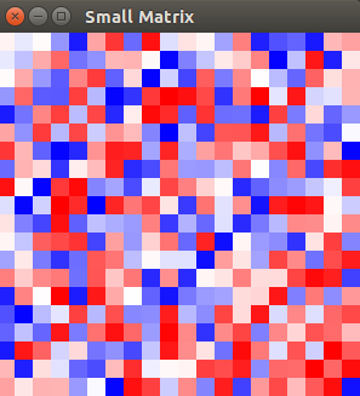# DMelt:Numeric/3 Random Matrices

Limitted access. First login to DataMelt if you are a full DataMelt member. Then login to HandWiki as a user.

# Random Matrices

A random matrix is a matrix filled with random variables. Read Random_matrix. The DataMelt contains many high-performance Java packages to construct random matrices.

## Using EJML

In this example we will illustrate the capabilities of the EJML library. Let us create a 20x20 matrix with random numbers between -10 and 10. The script below generates this matrix and visualize it:

This is the generated image. Block means an element is zero. Red positive and blue negative, while more intense the color larger the element's absolute value is.For more information how to generate random matrices, readRandomMatrices class.

## Using Parallel Colt

Matrix creation and manipulation can be fully multithreaded. In this approach, all processing cores of your computer will be used for calculations (or only a certain number of core as you have specified). Below we give a simple example. In the example below we create a large matrix 2000x2000 and calculate various characteristics of such matrix (cardinality, vectorize). We compare single threaded calculations with multithreaded ones (in this case, we set the number of cores to 2, but feel free to set to a large value).

To build random 2D matrices useDoubleFactory2D class. Here is a short example to create 1000x1000 matrix and fill it with random numbers:

from cern.colt.matrix        import *
from edu.emory.mathcs.utils  import ConcurrencyUtils
M=tdouble.DoubleFactory2D.dense.random(1000, 1000) # random matrix